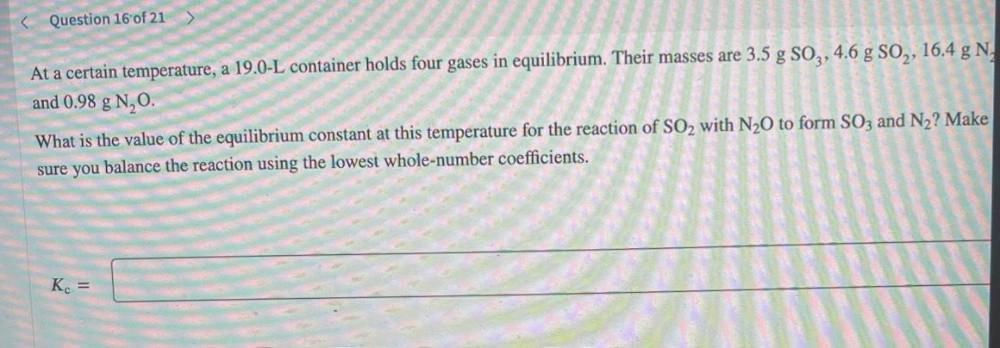Question:

# At a certain temperature, a 19.0-L container holds four gases in equilibrium. Their masses are 3.5 g SO3, 4.6 g SO₂, 16.4 g N, and 0.98 g N₂O. What is the value of the equilibrium constant at this temAt a certain temperature, a 19.0-L container holds four gases in equilibrium. Their masses are 3.5 g SO3, 4.6 g SO₂, 16.4 g N, and 0.98 g N₂O. What is the value of the equilibrium constant at this temperature for the reaction of SO2 with N₂O to form SO3 and N₂? Make sure you balance the reaction using the lowest whole-number coefficients. Kc=